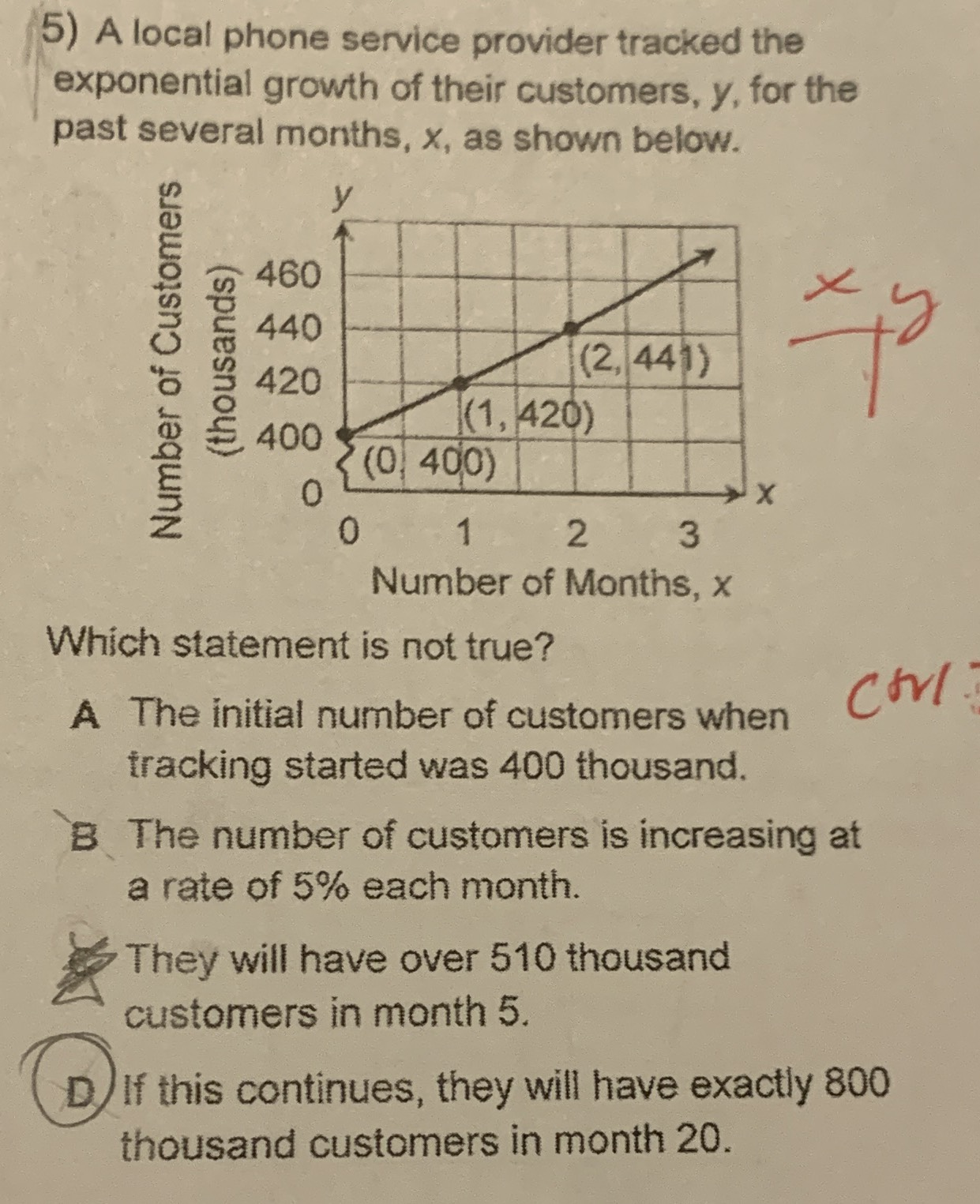### ¿Todavía tienes preguntas de matemáticas?

Pregunte a nuestros tutores expertos
Algebra
PreguntaA local phone service provider tracked the exponential growth of their customers, $$y$$ , for the past several months, $$x$$ , as shown below.

Which statement is not true?

A The initial number of customers when tracking started was $$400$$ thousand.

B The number of customers is increasing at a rate of $$5 \%$$ each month.

C. They will have over $$510$$ thousand customers in month $$5 .$$

D. If this continues, they will have exactly $$800$$ thousand customers in month $$20$$ .